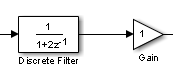Documentation

## How Propagation Affects Inherited Sample Times

During a model update, for example at the beginning of a simulation, Simulink® uses a process called sample time propagation to determine the sample times of blocks that inherit their sample times. The figure below illustrates a Discrete Filter block with a sample time period `Ts` driving a Gain block.Because the output of the Gain block is the input multiplied by a constant, its output changes at the same rate as the filter. In other words, the Gain block has an effective sample rate equal to the sample rate of the filter. The establishment of such effective rates is the fundamental mechanism behind sample time propagation in Simulink.

### Process for Sample Time Propagation

Simulink uses the following basic process to assign sample times to blocks that inherit their sample times:

1. Propagate known sample time information forward.

2. Propagate known sample time information backward.

3. Apply a set of heuristics to determine additional sample times.

4. Repeat until all sample times are known.

### Simulink Rules for Assigning Sample Times

A block having a block-based sample time inherits a sample time based on the sample times of the blocks connected to its inputs, and in accordance with the following rules:

RuleAction
All of the inputs have the same sample time and the block can accept that sample timeSimulink assigns the sample time to the block
The inputs have different discrete sample times and all of the input sample times are integer multiples of the fastest input sample timeSimulink assigns the sample time of the fastest input to the block . (This assignment assumes that the block can accept the fastest sample time.)
The inputs have different discrete sample times, some of the input sample times are not integer multiples of the fastest sample time, and the model uses a variable-step solverSimulink assigns a fixed-in-minor-step sample time to the block.
The inputs have different discrete sample times, some of the input sample times are not integer multiples of the fastest sample time, the model uses a fixed-step solver, and Simulink can compute the greatest common integer divisor (GCD) of the sample times coming into the blockSimulink assigns the GCD sample time to the block. Otherwise, Simulink assigns the fixed step size of the model to the block.
The sample times of some of the inputs are unknown, or if the block cannot accept the sample timeSimulink determines a sample time for the block based on a set of heuristics.